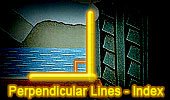Perpendicular Lines, Theorems and Problems - Table of Content  (5)Geometry Problem 1122. Triangle, 90 Degree, Perpendicular Lines, Orthocenter, Altitude, Midpoint, Collinear Points. Geometry Problem 1117. Right Triangle, Angle Trisection, 90 Degrees, Parallel Lines. Geometry Problem 1116. Right Triangle, Angle Trisection, 90 Degrees, Perpendicular Lines. Geometry Problem 1113. Triangle, Perpendicular Bisector, 90 Degrees, Circle, Concyclic Points, Cyclic Quadrilateral. Geometry Problem 1097. Quadrilateral, Inscribed Circle, 90 Degree, Angle, Area. Geometry Problem 1091. Square, Perpendicular, Distance, Area, Center. Geometry Problem 1090. Circle, Diameters, Chord, Perpendicular, Metric Relations. Geometry Problem 1088. Triangles, Perpendicular, Concurrent Lines. Go to Page: Previous | 1 | 2 | 3 | 4 | 5 | 6 | 7 | 8 | 9 | 10 | 11 | 12 | 13 | 14 | 15 | 16 | 17 | 18 | 19 | 20 | Next

 Home | Search | Geometry | Post a comment | Email | Post a comment | By Antonio Gutierrez Last updated: Feb 15, 2015